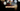# Leetcode | Solution of Contains Duplicate in JavaScript

March 31st, 2020
|

In this post, we will solve contains duplicate problem from leetcode and compute it's time & space complexity. Let's begin.

# Problem Statement

The question can be found at leetcode contains duplicate problem.

The problem states that we need to determine if there are any duplicates in a given array

# Solution

The problem is pretty simple. We can solve it in multiple ways

## Using Hashmap

We can iterate over the array and keep all the values in a map or object. In case the value already exists in the map, we have found our duplicate.

## Using Set

We will solve the question using a set here. Set only holds unique values. So if we add all the elements from the array into the set, the length of set and array should be equal if there are no duplicates.

We have discussed the approach, I urge you to go ahead on leetcode and give it another try.If you are here, it means something went wrong in implementation or you are just too lazy. In any case, let's see a simple implementation of the above logic.
``````var containsDuplicate = function(nums) {
const set = new Set(nums);

return set.size !== nums.length
};``````

Let's discuss the implementation.

On line 2, we are adding all the elements of the array to the Set. In the next line, we are checking if the length of the array is equal to the size of the set and return the answer accordingly.

This is all we need to solve the problem, once we submit it, these are the stats.

``````Status: Accepted
Runtime: 60ms
Memory: 40MB``````

## Time and space complexity

### Time complexity

We are adding all the numbers from the array into the set. So the complexity would be dependent on the implementation of Set. If I would have to take a guess, time complexity would be linear O(n).

### Space complexity

We are storing all the elements of the array in the set, so space complexity would be O(n).

# Summary

So, we solved the contains duplicate problem using a Set and calculated the time and space complexities.

I hope you enjoyed solving this question. This is it for this one, complete source code for this post can be found on my Github Repo. Will see you in the next one.